Home

# Relaxation of singular functionals defined on Sobolev spaces

## Abstract

In this paper, we consider a Borel measurable function on the space of $m\times n$matrices $f: M^{m\times n}\rightarrow \bar{\mathbb{R}}$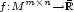taking the value $+\infty$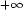, such that its rank-one-convex envelope $Rf$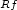is finite and satisfies for some fixed $p>1$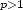: $$-c_0\leq Rf(F)\leq c(1+\Vert F\Vert^p)\ \hbox{for all}\ F\in M^{m\times n},$$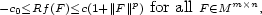where $c,c_0>0$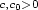. Let $\scriptstyle\O$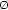be a given regular bounded open domain of $\mathbb{R}^n$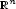. We define on $W^{1,p}(\O;\mathbb{R}^m)$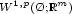the functional $$I(u)=\int_{\O}f(\nabla u(x))\ dx.$$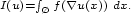Then, under some technical restrictions on $f$, we show that the relaxed functional $\scriptstyle\bar I$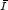for the weak topology of $W^{1,p}(\O;\mathbb{R}^m)$has the integral representation: $$\bar I(u)=\int_{\O}Q[Rf](\nabla u(x))\ dx,$$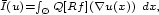where for a given function $g$, $Qg$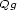denotes its quasiconvex envelope.

Hide All

# Relaxation of singular functionals defined on Sobolev spaces

## Metrics

### Full text viewsFull text views reflects the number of PDF downloads, PDFs sent to Google Drive, Dropbox and Kindle and HTML full text views.

Total number of HTML views: 0
Total number of PDF views: 0 *Loading metrics...

### Abstract viewsAbstract views reflect the number of visits to the article landing page.

Total abstract views: 0 *Loading metrics...

* Views captured on Cambridge Core between <date>. This data will be updated every 24 hours.

Usage data cannot currently be displayed.